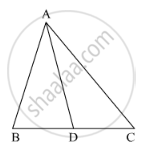Share

# In the Following Figure, Check Whether Ad is the Bisector of ∠A of ∆Abc in the Following Case: Ab = 5 Cm, Ac = 10 Cm, Bd = 1.5 Cm and Cd = 3.5 Cm - CBSE Class 10 - Mathematics

#### Question

In the following figure, check whether AD is the bisector of ∠A of ∆ABC in the following case:

AB = 5 cm, AC = 10 cm, BD = 1.5 cm and CD = 3.5 cm#### Solution

It is given that AB = 5cm, AC= 10cm, BD = 1.5cm and CD = 3.5cm.

We have to check whether AD is bisector of ∠A.

First we will check proportional ratio between sides.

Now

"AB"/"AC"=5/10=1/2

"BD"/"CD"=1.5/3.5=3/7

Since "AB"/"AC"!="BD"/"CD"

Hence AD is not the bisector of ∠A.

Is there an error in this question or solution?

#### Video TutorialsVIEW ALL 

Solution In the Following Figure, Check Whether Ad is the Bisector of ∠A of ∆Abc in the Following Case: Ab = 5 Cm, Ac = 10 Cm, Bd = 1.5 Cm and Cd = 3.5 Cm Concept: Angle Bisector.
S